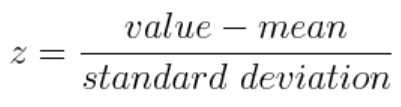# Zakaria Jaadi - «A step by step explanation of Principal Component Analysis» (2019-02-28)

## So what is Principal Component Analysis?

Principal Component Analysis, or PCA, is a dimensionality-reduction method that is often used to reduce the dimensionality of large data sets, by transforming a large set of variables into a smaller one that still contains most of the information in the large set.

Reducing the number of variables of a data set naturally comes at the expense of accuracy, but the trick in dimensionality reduction is to trade a little accuracy for simplicity because:

• smaller data sets are easier to explore and visualize
• make analyzing data much easier and faster for machine learning algorithms without extraneous variables to process.

## Step 1. Standardization

The aim of this step is to standardize the range of the continuous initial variables so that each one of them contributes equally to the analysis.

More specifically, the reason why it is critical to perform standardization prior to PCA, is that the latter is quite sensitive regarding the variances of the initial variables.

That is, if there are large differences between the ranges of initial variables, those variables with larger ranges will dominate over those with small ranges (for example, a variable that ranges between 0 and 100 will dominate over a variable that ranges between 0 and 1), which will lead to biased results.
So, transforming the data to comparable scales can prevent this problem.

Mathematically, this can be done by subtracting the mean and dividing by the standard deviation for each value of each variable.Once the standardization is done, all the variables will be transformed to the same scale.

## Step 2. Covariance matrix computation

towardsdatascience.com/a-step-by-step-explanation-of-principal-component-analysis-b836fb9c97e2